Tuesday 29th September 2020

CBSE Guess > Papers > Question Papers > Class XII > 2003 > Chemistry > Delhi Set -II

CHEMISTRY—2003 (Set II—Delhi)

Note: Except for the following questions, all the remaining questions have been asked in Set I.

Q. 4. Why is the equilibrium constant K related to only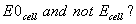1

Q. 6. Write IUPAC name of the complex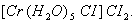1

Q. 7. How is ammonia molecule a good ligand? 1

Q. 8. Complete the nuclear equation: 1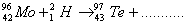Q. 15. A piece of wood was found to have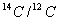ratio 0.7 times then that in the living plant. Calculate the period when the piece of wood separated from the living plant. 2
[t1/2 for 14C = 5760 years]

Q. 21. Using the valence bond approach, predict the shape and magnetic character of 2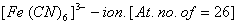Q. 22. Write one chemical reaction each to exemplify the following:
(i) Cannizzaro reaction
(ii) Williamson's synthesis 2

Q. 25. Account for the following:
(a) Zirconium and Hafnium exhibit almost similar properties.
(b) Zinc salts are white whilesalts are coloured. [At. nos. Zn = 30, Cu = 29] 2

Q. 29. How is the third law of thermodynamics useful in calculation of the absolute entropies? Calculate the value offor the following reaction at 400 K:If the value of equilibrium constant for the reaction at 400 K is 1.958 x 10 -4 and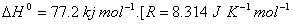Q. 30. Write chemical tests to distinguish between: 3
(i) Acetaldehyde and Acetone
(ii) Acetic acid and Acetaldehyde
(iii) Phenol and Propanoic acid

Q. 31. Calculate the cell emf at 25° C for the following cell: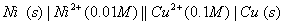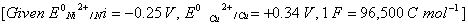Calculate the maximum work that can be accomplished by the operation of this cell. 3

 Chemistry 2003 Question Papers Class XII Delhi Outside Delhi Compartment Delhi Compartment Outside DelhiSet ISet ISet ISet ISet IISet IISet IISet II

CBSE 2003 Question Papers Class XII